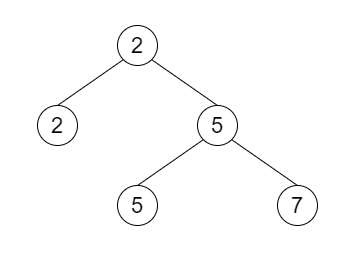# Second Minimum Node In a Binary Tree in C++

Suppose there is a non-empty special binary tree with some non-negative value, here each node in this tree has exactly two or zero children. If the node has two children, then this node's value is the smaller value among its two children. In other words, we can say that [root.val = minimum of root.left.val, root.right.val]. If we have such binary tree, we have to find the second minimum value in the set made of all the nodes' value in the whole tree. If there is no such element, then return -1 instead.

So, if the input is likethen the output will be 5. The smallest value is 2, the second smallest value is 5.

To solve this, we will follow these steps −

• Define a function TraverseNodes(), this will take node, min, nextMin,
• if node is null, then −
• return
• if val of node > min, then −
• if nextMin is same as -1 or val of node < nextMin, then −
• nextMin := val of node
• TraverseNodes(left of node, min, nextMin)
• TraverseNodes(right of node, min, nextMin)
• From the main method do the following −
• min := value of root when root is not null, otherwise -1
• nextMin := -1
• TraverseNodes(root, min, nextMin)
• return nextMin

Let us see the following implementation to get better understanding −

## Example

Live Demo

#include <bits/stdc++.h>
using namespace std;
class TreeNode{
public:
int val;
TreeNode *left, *right;
TreeNode(int data){
val = data;
left = NULL;
right = NULL;
}
};
void insert(TreeNode **root, int val){
queue<TreeNode*> q;
q.push(*root);
while(q.size()){
TreeNode *temp = q.front();
q.pop();
if(!temp->left){
if(val != NULL)
temp->left = new TreeNode(val);
else
temp->left = new TreeNode(0);
return;
}else{
q.push(temp->left);
}
if(!temp->right){
if(val != NULL)
temp->right = new TreeNode(val);
else
temp->right = new TreeNode(0);
return;
}else{
q.push(temp->right);
}
}
}
TreeNode *make_tree(vector<int> v){
TreeNode *root = new TreeNode(v);
for(int i = 1; i<v.size(); i++){
insert(&root, v[i]);
}
return root;
}
class Solution {
public:
int findSecondMinimumValue(TreeNode* root) {
int min = (root && root->val != 0) ? root->val : -1;
int nextMin = -1;
TraverseNodes(root, min, nextMin);
return nextMin;
}
void TraverseNodes(TreeNode* node, int min, int& nextMin) {
if (!node || node->val == 0) {
return;
}
if (node->val > min) {
if (nextMin == -1 || node->val < nextMin) {
nextMin = node->val;
}
}
TraverseNodes(node->left, min, nextMin);
TraverseNodes(node->right, min, nextMin);
}
};
main(){
Solution ob;
vector<int> v = {2,2,5,NULL,NULL,5,7};
TreeNode *root = make_tree(v);
cout << (ob.findSecondMinimumValue(root));
}

## Input

{2,2,5,NULL,NULL,5,7}

## Output

5